Question

Four long parallel wires each carry a current of 1.0 A. The current direction is out...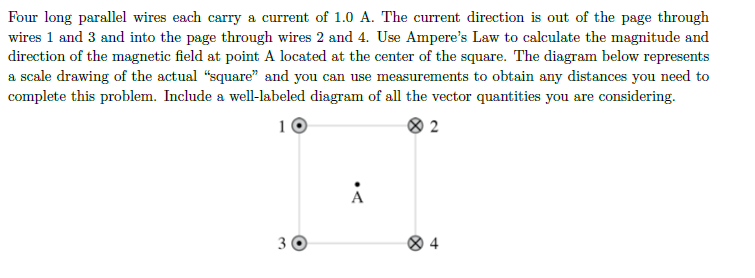Four long parallel wires each carry a current of 1.0 A. The current direction is out of the page through wires 1 and 3 and into the page through wires 2 and 4. Use Ampere's Law to calculate the magnitude and direction of the magnetic field at point A located at the center of the square. The diagram below represents a scale drawing of the actual "square" and you can use measurements to obtain any distances you need to complete this problem. Include a well-labeled diagram of all the vector quantities you are considering.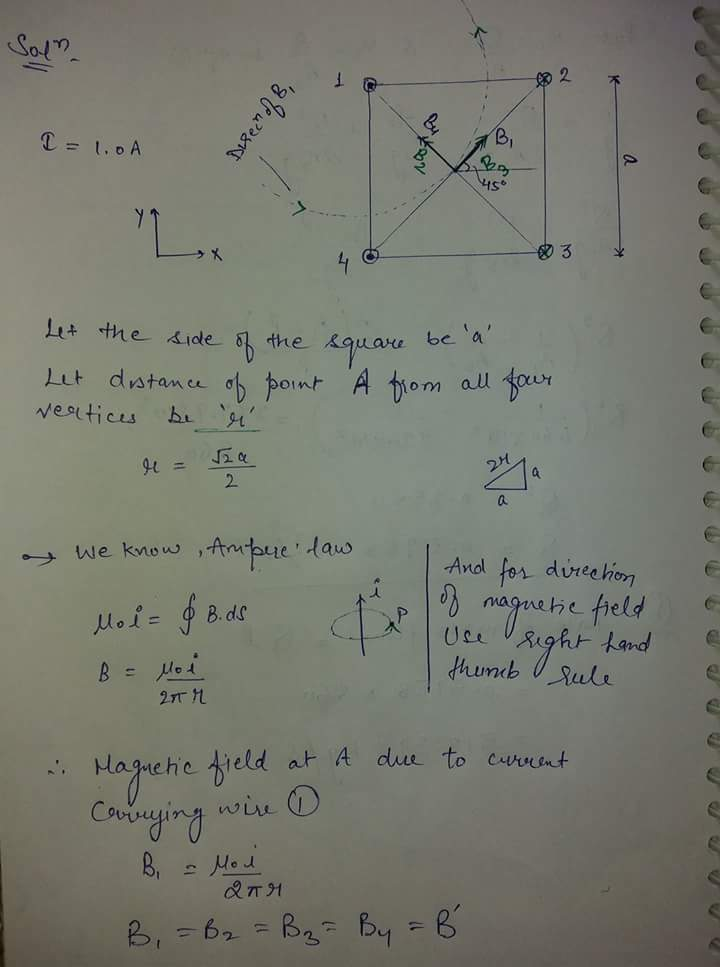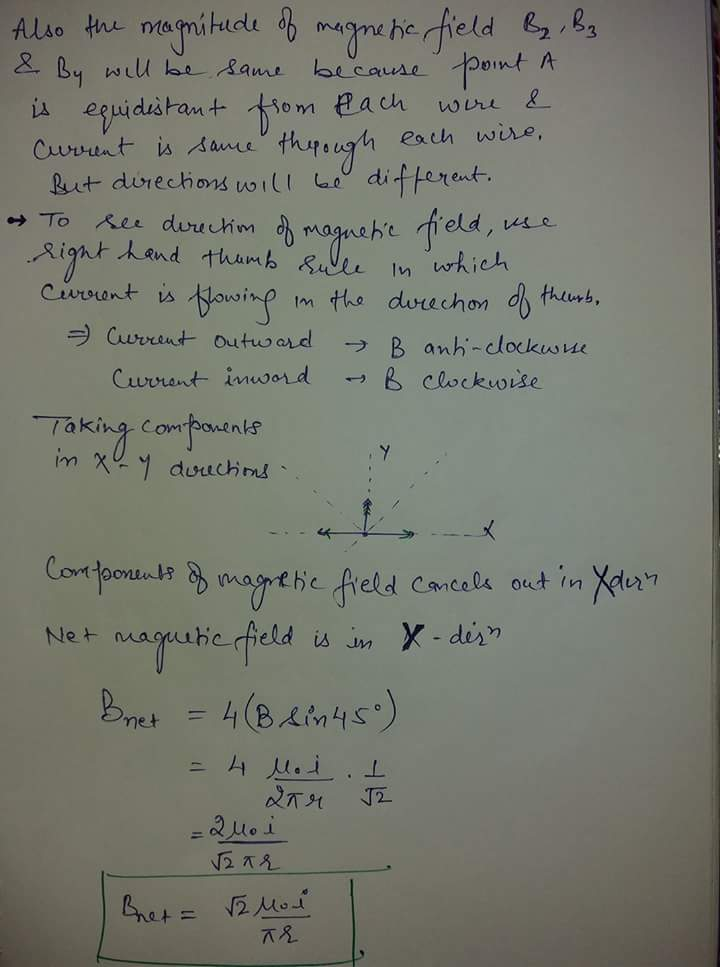Earn Coins

Coins can be redeemed for fabulous gifts.

Similar Homework Help Questions
• Four long parallel wires each carry a 2.0 A current in the same direction. The wires...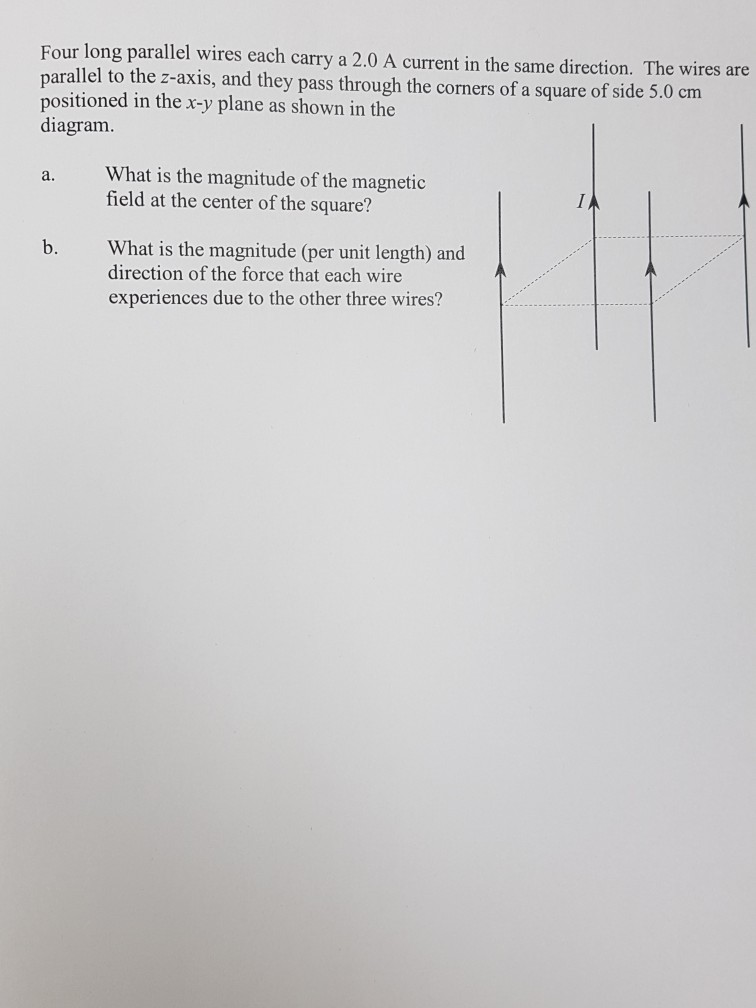Four long parallel wires each carry a 2.0 A current in the same direction. The wires are parallel to the z-axis, and they pass through the corners of a square of side 5.0 cm in the x-y plane as shown in the diagram. What is the magnitude of the magnetic field at the center of the square? a. b. What is the magnitude (per unit length) and direction of the force that each wire experiences due to the other three...

• Two long, parallel wires carry a current of 4.45 A in a direction that is out...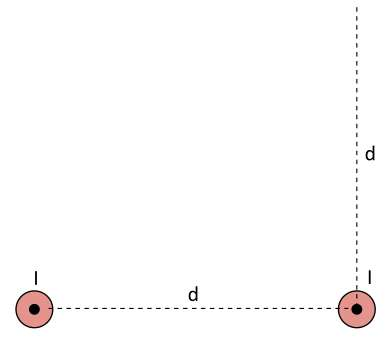Two long, parallel wires carry a current of 4.45 A in a direction that is out of the page. The wires are d = 5.33 cm apart. Determine the magnitude of the net magnetic field, in ?T, at a point that is the same distance d above one of the wires as shown. (You need to determine the vector sum of the two magnetic fields.) Answer: 26.4 microT

• Two long, parallel wires carry a current of 5.00 A in a direction that is out...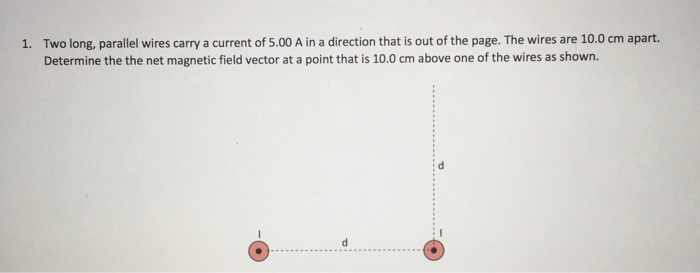Two long, parallel wires carry a current of 5.00 A in a direction that is out of the page. The wires are 10.0 cm apart. Determine the the net magnetic field vector at a point that is 10.0 cm above one of the wires as shown. 1.

• Three long straight wires are parallel to each other and carry currents directed into or out...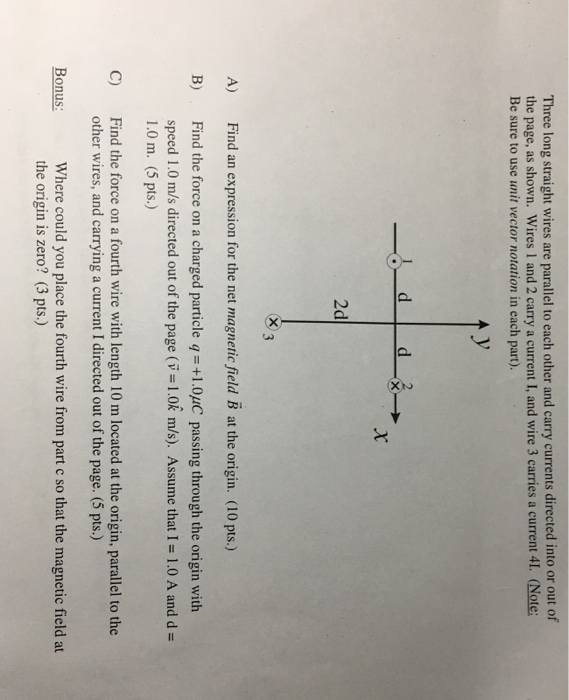Three long straight wires are parallel to each other and carry currents directed into or out of the page, as shown. Wires 1 and 2 carry a current I, and wire 3 carries a current 41. (Note: Be sure to use unit vector notation in each part). 2d indn rssio for th net magnaie fald B ) B) Find the force on a charged particle q-+1.0uC passing through the origin with speed 1.0 m/s directed out of the page (v-1.0k...

• Four long parallel wires each carry 2.0 A in the same direction. They are parallel to...

Four long parallel wires each carry 2.0 A in the same direction. They are parallel to the z-axis, and they pass through the corners of a square of side 4.0 cm positioned in the x-y plane. What net magnetic field does one of the wires experience due to the other wires?

• Problem 1 Three long straight wires are parallel to each other and carry currents directed into...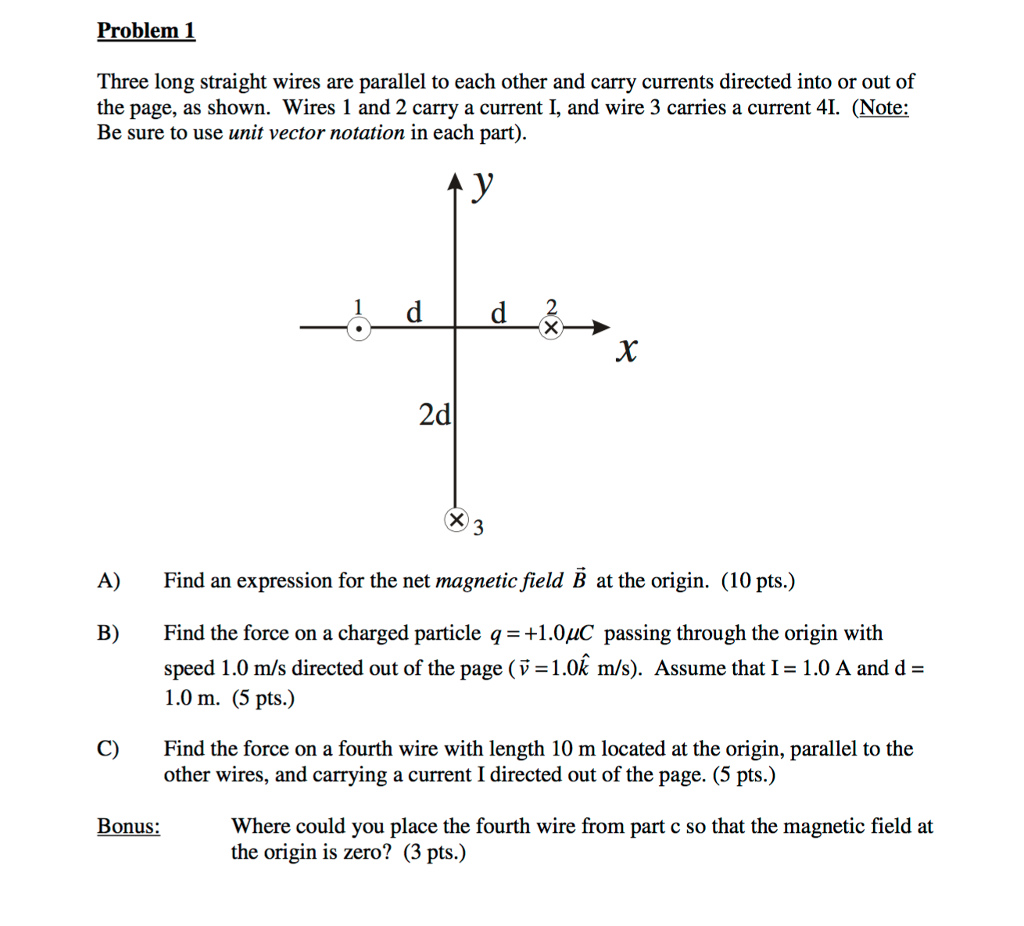Problem 1 Three long straight wires are parallel to each other and carry currents directed into or out of the page, as shown. Wires 1 and 2 carry a current I, and wire 3 carries a current 4. (Note: Be sure to use unit vector notation in each part) 2 2d A) Find an expression for the net magnetic field B at the origin. (10 pts.) B) Find the force on a charged particle q1.0uC passing through the origin with...

• PLEASE HELP!!! 8. Two long, parallel wires each carry a current I in the same direction...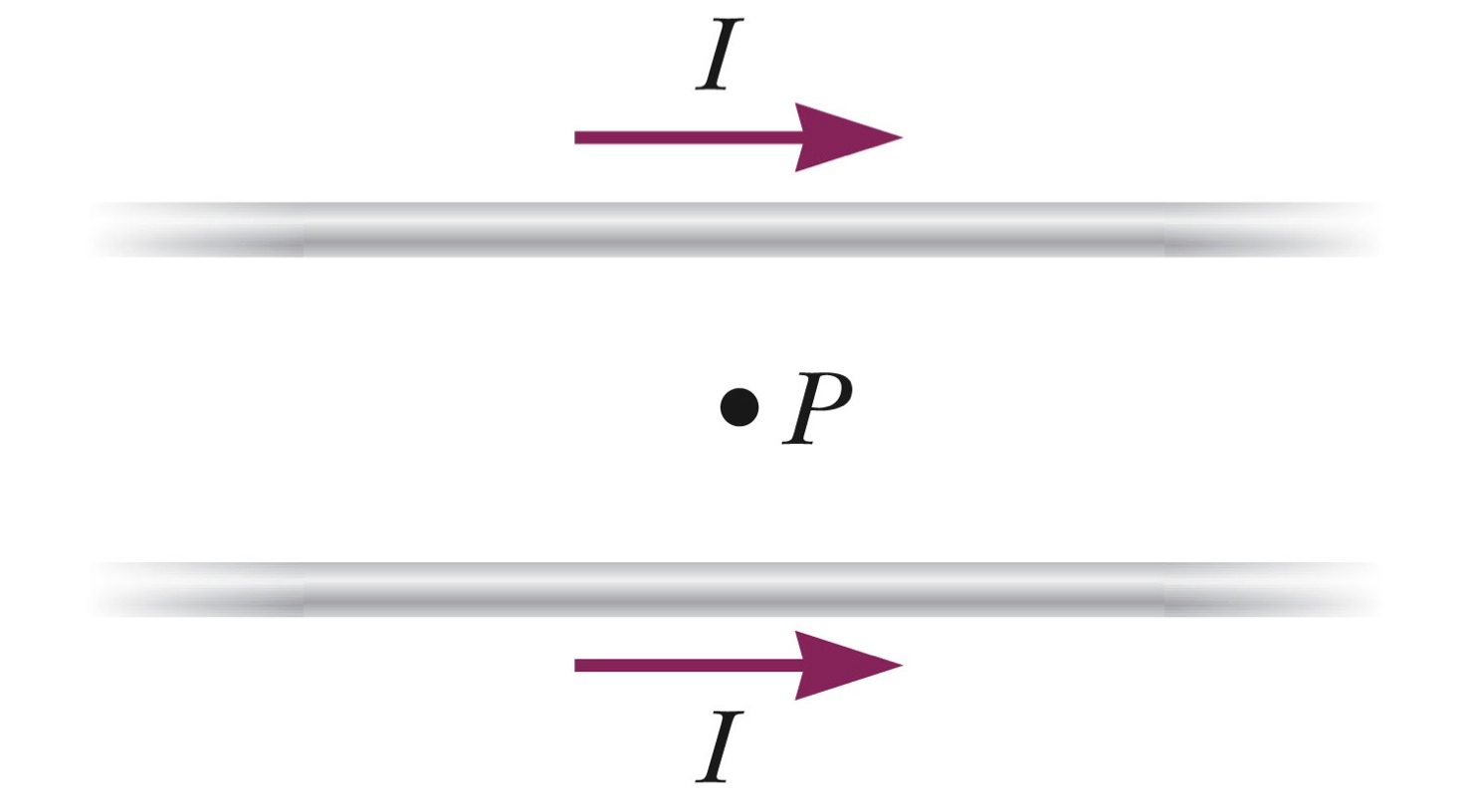PLEASE HELP!!! 8. Two long, parallel wires each carry a current I in the same direction as shown in the figure. The magnetic field at the point P, midway between the two wires is ________. zero directed into the page directed out of the page parallel to the wires in the same direction as the current. parallel to the wires, in the opposite direction as the current. 9. A long, straight wire carries a current of 20A to the right....

• Three long wires, all parallel to each other, each carry a current of 10 A out...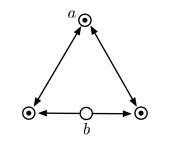Three long wires, all parallel to each other, each carry a current of 10 A out of the page. The wires sit on the vertices of an equilateral triangle with sides of 12.5 cm, as shown below. A fourth wire (wire b) passes through the midpoint of the base of the triangle and is parallel to the other three wires. What is the net magnetic force on wire a (the top vertex of the triangle) if wire b carries a...

• Four long, parallel conductors carry equal currents of I2.60 A. The figure below is an end view of the conductors. The current direction is into the page at points A and B and out of the page at C an...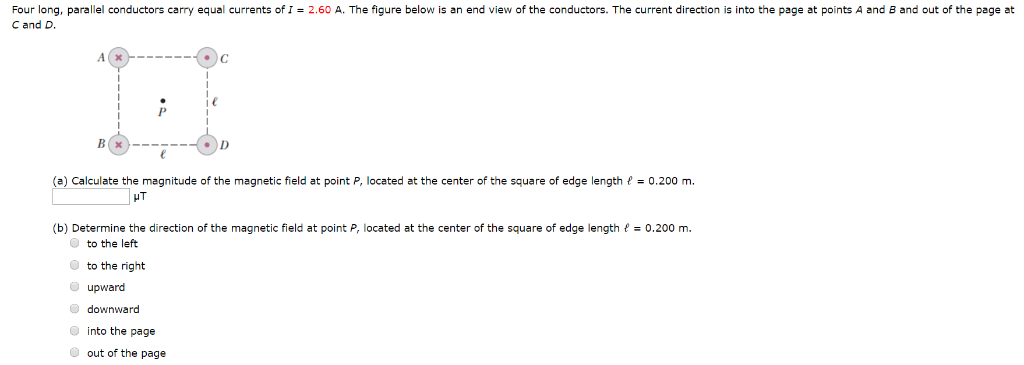Four long, parallel conductors carry equal currents of I2.60 A. The figure below is an end view of the conductors. The current direction is into the page at points A and B and out of the page at C and D (a) Calculate the magnitude of the magnetic field at point P, located at the center of the square of edge length f = 0.200 m. HT (b) Determine the direction of the magnetic field at point P, located at...

• Three very long, straight parallel wires each carry currents of I, directed out of the page....

Three very long, straight parallel wires each carry currents of I, directed out of the page. The wires pass through the vertices of a right isosceles triangle of side d. What is the magnitude of the magnetic field at a point P at the midpoint of the hypotenuse of the triangle? There is no diagram.